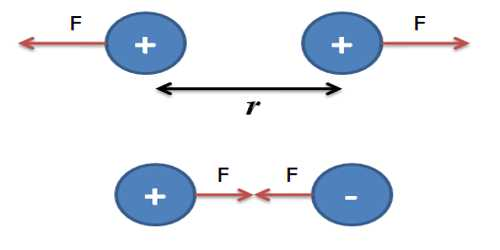# Coulomb’s Law in terms of Point Charges

Coulomb’s Law in terms of Point Charges

We know, a charge attracts or repels another charge. The force of attraction or repulsion between two charges depends on the following three factors, viz.-

(i) Amount of two charges

(ii) Distance between the two charges and

(iii) The medium between the two charges.

Based on the above conditions, in 1787, French scientist Coulomb gave law acting between two charges. It is known as Coulomb’s law. Before starting the law, we need to know about point charge. Because Coulomb’s law is applicable only to the point charge. That means this law is applicable only to those bodies whose sizes are extremely small compared to the distance between the charges. By applying Coulomb’s law directly the force between two extended charged bodies cannot be determined. There is a similarity of the force between the two charges and similar terms relating magnet and gravity.

Point charge: When the size of the charged body is extremely small, then that charged body is called point charge. Those charged bodies are so small compared to the distances between them that they may be considered as mathematical points.

Now, we define Coulomb’s law with the help of point charges.

Coulomb’s law: At a particular medium, the magnitude of the attractive or repulsive force between two point charges is directly proportional to the product of the charges and inversely proportional to the square of the distance between the charges. The force acts along the straight line joining the two charges.